## Tuesday, March 8, 2011

### Ishaka's Great Big Book Of Integers

Review

- zero pair -a positive # and a negative # combined make a zero pair.
(Eg. 1-1=0)

- number lines can be used.

- "When subtracting something th
at isn't there, use a zero pair." This saying helps when subtracting integers."

- Gr. 7 way:
(+7) + (-3) =
positive 7 and negative 3.
have 7 and owe 3.
+7 + -3

Gr. 8 way:
7 - 3 = 4

-Stared Homework Questions:

-3 - (-7) = 4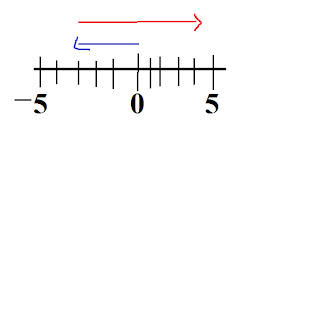-3 - 7 = -103 - 7 = -4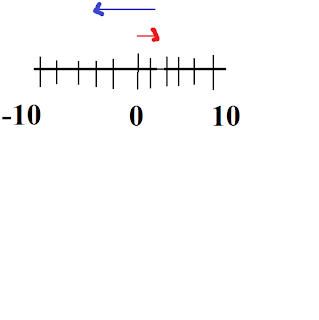3 + 7 = 10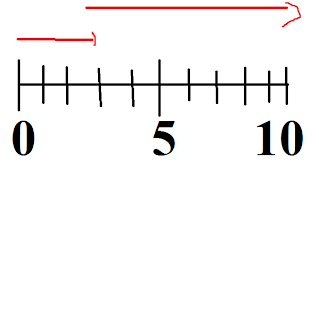-3 + 7 = 4Chapter 2: Multiplying Integers

- Sign Rule (negative signs)
Whenever you have an odd number of negative factors the product will be a NEGATIVE number, and when you have an even number of negative factors the product will be a POSITIVE number.

(+2) x (+3)= 6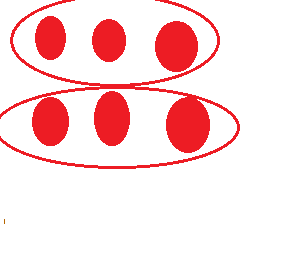(+2) x (-3)= -6(-2) x (+3)= -6(-2) x (-3)= 6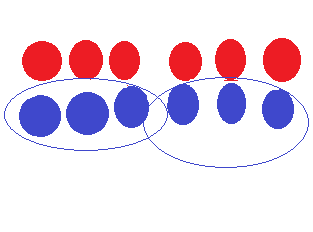Chapter 3: Dividing Integers
Partitive Division: means how many groups there are and how many integers are in that group

6÷ 2=3
means in partitive division-
How many groups of +2 are in 6?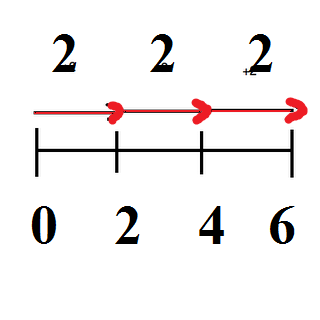-6 ÷ (-2)= 3
means in partitive division-
How many groups of -2 are in -6?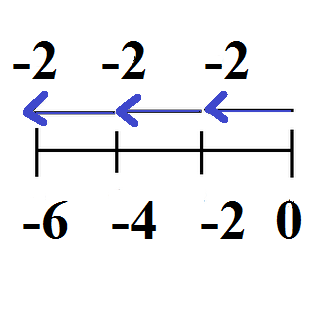Quotative Division
: means sharing equally so that every group has the same amount of integers

(-6) ÷ 2 = -3
Share (-6) with 2 groups.Multiplicative Division

-6 ÷ -2 = 3
-3 x -2 = 6
-2 x -3 = 6

-6 ÷ 2 = -3
2 x -3 = -6
-3 x 2 = -6

(+5) x (-3) + (-6) ÷ (+3)=

[(+5) x (-3)] + [(-6) ÷ (+3)]=

(-15) + (-18) = +33

Chapter 4: Order Of Operations With Integers

BEDMAS: Brackets, Exponents, Division, Multiplication, Addition, Subtraction

(+10) x (-5) + (+6) ÷ (+3)

[(+10) x (-5)] + [(+6) - (+3)]

2. answer what's in the square brackets

[(+10) x (-5)] + (+6) - (+3)
-50 +

3. After the brackets you find the exponents
4. Then after exponents do the multiplication and division (answer left - right)

[(+10) x (-5)] + (+6) - (+3)
-50 +
-50 + 2
5. After the multiplication/ division then you do add and subtract (answer left - right)